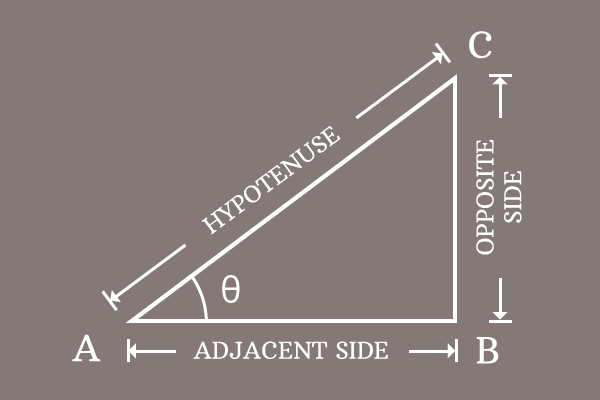# Quotient identity of Sine and Cosine with Tangent

The quotient relation of sine and cosine functions with tangent function is called the quotient identity of sine and cosine functions with tangent function.

## Formula

$\dfrac{\sin{\theta}}{\cos{\theta}} \,=\, \tan{\theta}$

### Introduction

Sine function can be divided by the cosine function and the quotient of them represents another trigonometric function tangent. The quotient relation of sine and cosine functions with tangent function is used as basic trigonometric formula in mathematics.

#### Proof

$\Delta BAC$ is a right angled triangle, whose angle is assumed as theta ($\theta$).##### Define Sine and Cosine functions

Express sine and cosine functions in terms of ratio of the sides of the right angled triangle $BAC$.

$\sin{\theta} \,=\, \dfrac{Length \, of \, Opposite \, side}{Length \, of \, Hypotenuse} \,=\, \dfrac{BC}{AC}$

$\cos{\theta} \,=\, \dfrac{Length \, of \, Adjacent \, side}{Length \, of \, Hypotenuse} \,=\, \dfrac{AB}{AC}$

##### Divide Sine function by Cosine

Divide sine function by cosine function and simplify it to obtain quotient of them.

$\dfrac{\sin{\theta}}{\cos{\theta}} \,=\, \dfrac{\dfrac{BC}{AC}}{\dfrac{AB}{AC}}$

$\implies$ $\dfrac{\sin{\theta}}{\cos{\theta}}$ $\,=\,$ $\dfrac{BC}{AC} \times \dfrac{AC}{AB}$

$\implies$ $\dfrac{\sin{\theta}}{\cos{\theta}}$ $\,=\,$ $\dfrac{BC}{AB} \times \dfrac{AC}{AC}$

$\implies$ $\dfrac{\sin{\theta}}{\cos{\theta}}$ $\,=\,$ $\dfrac{BC}{AB} \times \require{cancel} \dfrac{\cancel{AC}}{\cancel{AC}}$

$\implies$ $\dfrac{\sin{\theta}}{\cos{\theta}}$ $\,=\,$ $\dfrac{BC}{AB} \times 1$

$\implies$ $\dfrac{\sin{\theta}}{\cos{\theta}}$ $\,=\,$ $\dfrac{BC}{AB}$

##### Expressing quotient of sine and cosine functions

The quotient of sine by cosine function is a ratio of two sides. Actually, the ratio of length of opposite side ($BC$) by length of adjacent side ($AB$) represents tangent function as per the fundamental definition of the tangent function.

$\tan{\theta} \,=\, \dfrac{Length \, of \, Opposite \, side}{Length \, of \, Adjacent \, side}$

$\implies \tan{\theta} \,=\, \dfrac{BC}{AB}$

Therefore, it is proved that the quotient of sine function by cosine function is equal to the tangent function mathematically.

$\,\,\, \therefore \,\,\,\,\,\, \dfrac{\sin{\theta}}{\cos{\theta}} \,=\, \tan{\theta}$

Email subscription
Math Doubts is a best place to learn mathematics and from basics to advanced scientific level for students, teachers and researchers. Know more
Follow us on Social Media
###### Math Problems

Learn how to solve easy to difficult mathematics problems of all topics in various methods with step by step process and also maths questions for practising.

Learn more